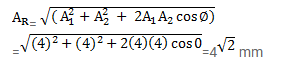# Two waves, travelling in the same direction through the same region,Question:

Two waves, travelling in the same direction through the same region, have equal frequencies, wavelengths and amplitudes. If the amplitude pf each wave is $4 \mathrm{~mm}$ and the phase difference between the waves is $90^{\circ}$, what is the resultant amplitude?

Solution: